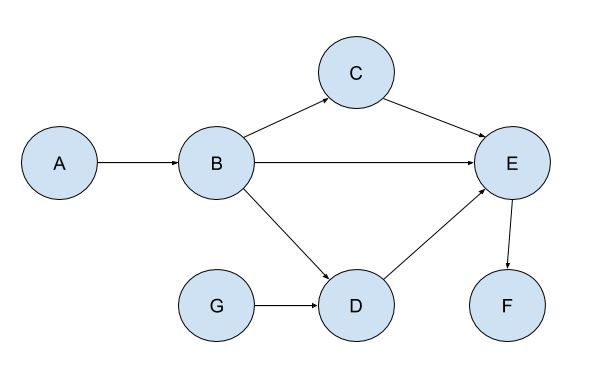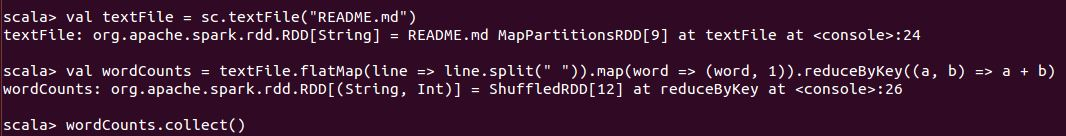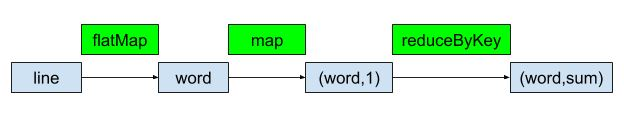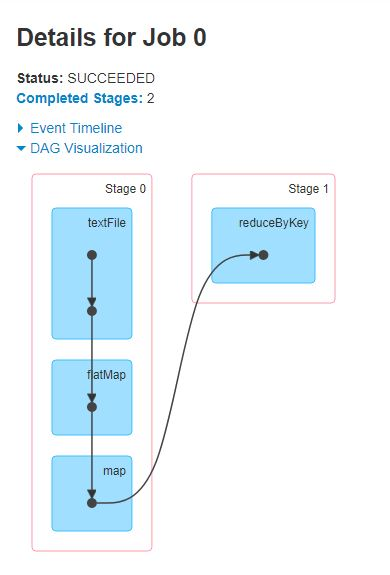Introduction

In the article, Apache Spark 1, I looked at a high overview of what Apache Spark is and its architecture. I touched on the fact that at the core of Apache Spark there is a very advanced Direct Acyclic Graph (DAG) data processing engine. In this article I will look into DAG and how it works in Spark.

What is Direct Acyclic Graph (DAG) ?

Spark is efficient due to its advanced Directed Acyclic Graph (DAG) data processing engine. To understand how DAG works, we have to break it down and define each word.

Graph, what does it mean? Its a diagram representing a system of connections or interrelations among two or more things by a number of distinctive dots, lines, bars and so forth. In our case, which is DAG, the graph is a representation of connected nodes and they are connected by what are know as edges.

Acyclic, what does it mean?  Its an adjective that describes a graph in which there is no cycle or closed path.

Directed, what does it mean? In the context of DAG, its a direction an edge has to take and it is represented by an arrow.From the diagram above, we have a graph with a collection of nodes denoted by the letters A to F and they are connected by edges which have specific directions, hence the arrows. If we are to follow the movement, say from A, we will never go back to A, hence they are acyclic. There is no circular movement and because of that, it enables dependency tracking, for instance, B depends on A and F on E and so forth. If we think of it as a data processing pipeline, A and G will be the source of the data and it will go through several transformation steps until it gets to F which will be the final result.

Now in the context of Spark, our nodes are RDDs and the arrows are Transformations. Below is an example of how DAG works in Spark:This is a simple word count that will be done for a ReadMe file. Visually the process will look as follows:The textFile is our first RDD whose element is a line and it will be transformed using flatMap to another RDD whose element is a word and then map  will transform the word to a key value pair RDD. The reduceByKey will then do the summation of the counts of each word. Spark comes with its own DAG visualisation tool. From that tool the DAG diagram will look as follows:Now, if we look at the first DAG diagram, whereby we were using letters as nodes, we established that letter A and G will represent the source of our data. The final result will then be letter F, after it has gone through some transformation. This is the same with the above example, the ReadMe file is the source and as it moves from Stage 0 to Stage 1, the data will go through some transformation. But then one would ask, why the split between Stage 0 and 1? Why is reduceByKey in its own stage?

As I mentioned earlier, one of the advantages of DAG is the tracking of dependencies. The textFile has to be flatMapped -> mapped -> reducedByKey.  Each line from the ReadMe file will be transformed to words. Now each word does not have any dependency on the other words. However, when we now want to find the number of occurrences of each word, there is going to be need of movement of words. In Spark, this is known as shuffling and if there is need for shuffling, Spark sets that as a boundary between stages. If a task will result in shuffling, it will be placed in its own stage, hence, reduceByKey is in Stage 1. Once that is done, tasks in each stage are then bundled together and are sent to the executors.

Conclusion

In this article, I managed to explain what DAG is in Spark context and also gave an example on how it works in Spark. This is just scratching the surface since more is involved when running a Spark application. For instance, when a Spark application is submitted to Apache Spark, one needs to understand how to set Spark configurations for Spark to work efficiently. Therefore, questions like, how much driver memory should I use, how many executors or number of cores per each executor do I need, will need to be answered.

When developing a Spark application, one also need to understand what transformation is and what action is in the context of Spark. Understanding these terms will help in developing an application that will be executed efficiently.

Will try to cover these concepts in the next articles on Apache Spark.

Source
Acyclic
DAG
Execution plan